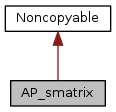ARB
AP_smatrix Class Reference

`#include <AP_matrix.hxx>`

Inheritance diagram for AP_smatrix:[legend]
Collaboration diagram for AP_smatrix:[legend]

## Public Member Functions

AP_smatrix (size_t si)

~AP_smatrix ()

void set (size_t i, size_t j, AP_FLOAT val)

AP_FLOAT fast_get (size_t i, size_t j) const

AP_FLOAT get (size_t i, size_t j) const

AP_FLOAT get_max_value () const

size_t size () const

## Detailed Description

Definition at line 32 of file AP_matrix.hxx.

## Constructor & Destructor Documentation

 AP_smatrix::AP_smatrix ( size_t si )
explicit

Definition at line 24 of file AP_matrix.cxx.

 AP_smatrix::~AP_smatrix ( )

Definition at line 39 of file AP_matrix.cxx.

## Member Function Documentation

 void AP_smatrix::set ( size_t i, size_t j, AP_FLOAT val )
inline

Definition at line 43 of file AP_matrix.hxx.

 AP_FLOAT AP_smatrix::fast_get ( size_t i, size_t j ) const
inline

Definition at line 45 of file AP_matrix.hxx.

References ap_assert.

Referenced by PH_NEIGHBOURJOINING::PH_NEIGHBOURJOINING().

 AP_FLOAT AP_smatrix::get ( size_t i, size_t j ) const
inline

Definition at line 46 of file AP_matrix.hxx.

Referenced by DI_MATRIX::compress(), di_mark_by_distance(), and DI_MATRIX::save().

 AP_FLOAT AP_smatrix::get_max_value ( ) const

Definition at line 43 of file AP_matrix.cxx.

References max.

Referenced by PH_NEIGHBOURJOINING::PH_NEIGHBOURJOINING().

 size_t AP_smatrix::size ( ) const
inline

Definition at line 50 of file AP_matrix.hxx.

The documentation for this class was generated from the following files: